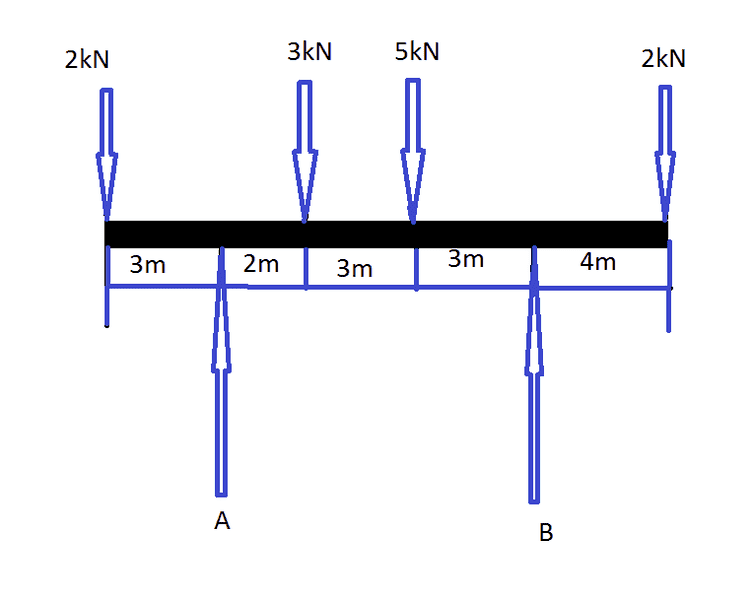# Moments of Force

1.How do I find the force of A and B. I am taking moments at A but I am not getting the correct answer. (More Details below of attempt)

## Homework Equations

Ʃ Of Clockwise Moments = Ʃ Of Anti-Clockwise Moments
Ʃ Of Upward Force = Ʃ Of Downward Force

## The Attempt at a Solution

(THIS IS WRONG BUT THIS IS MY ATTEMPT)

A => (3x2)+(5x5)+(12x2)= Bx11
=> 6 + 25 + 24 = B x 11
=> 55 = B x 11
=> 55/11 = B
=> 5 = b

THANKS FOR HELP, I was trying this weekend and I need some genius advice so when I get this question again, I will be able to do so :)